Subtotal: - This option is used to calculate rows of related data quickly by inserting subtotals and totals.Let’s take an example to understand how we can use the Subtotal in Microsoft Excel.

We have data in range A1:E18, column A contains month, column B contains year, column C contains Date, column D contains Customer name and column E contain Revenue (\$).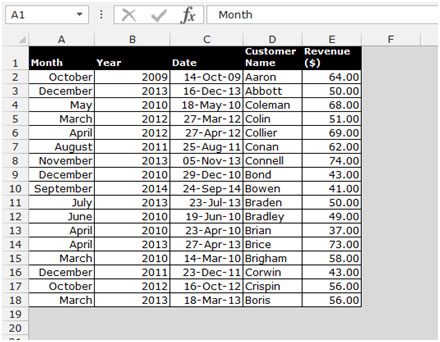• Sort the data as per the year, newest to oldest.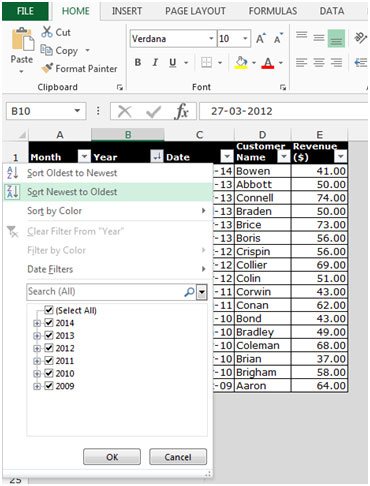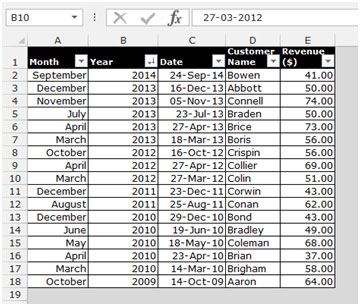To add the subtotal to a Date field, follow below given steps:-

• Go to data Tab, Select Subtotal from the Outline group.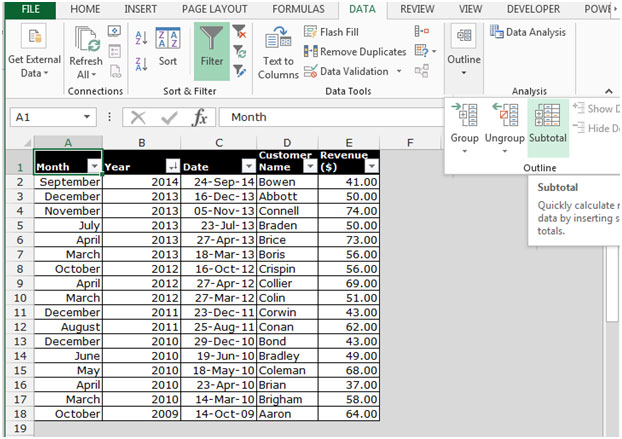• Subtotal dialog box will appear.
• Select year from the drop down list of At each change in.
• To add the fields, select Sum from the function drop down list.
• Select Revenue, click on OK.• The data will get add as per the date field in Microsoft Excel.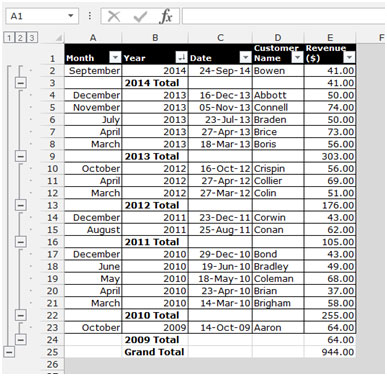• From the data menu, select Subtotal from the Outline group.
• Subtotal dialog box will appear.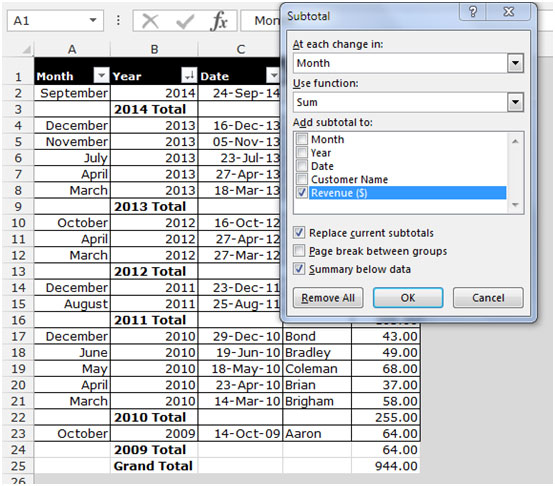• From the Use function, select a different function.
• Deselect the replace current subtotals checkbox and then click OK.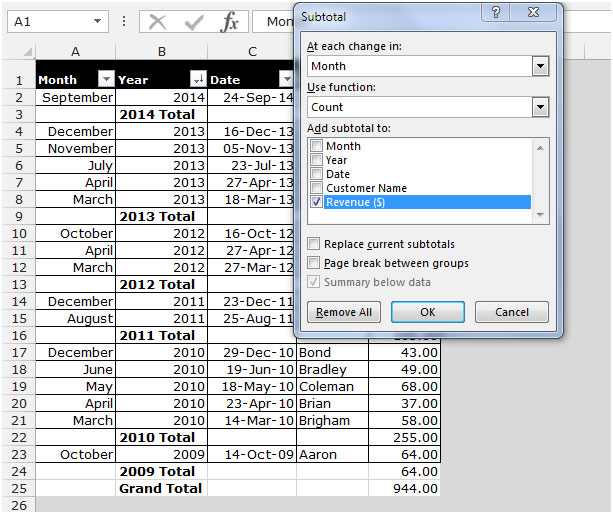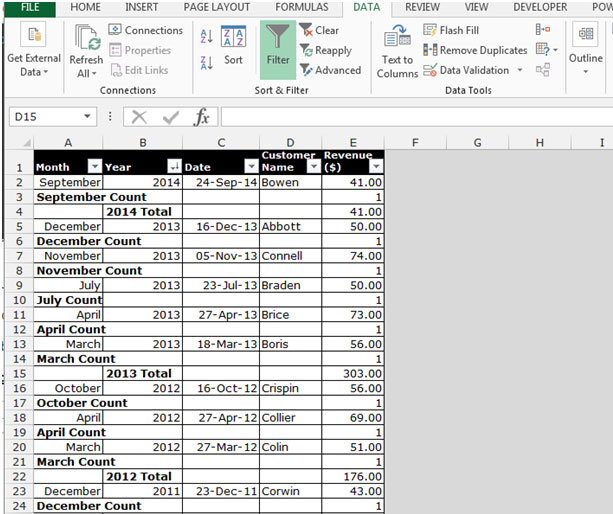• If you want to add more subtotals repeat the same steps to add more subtotals function to the list.